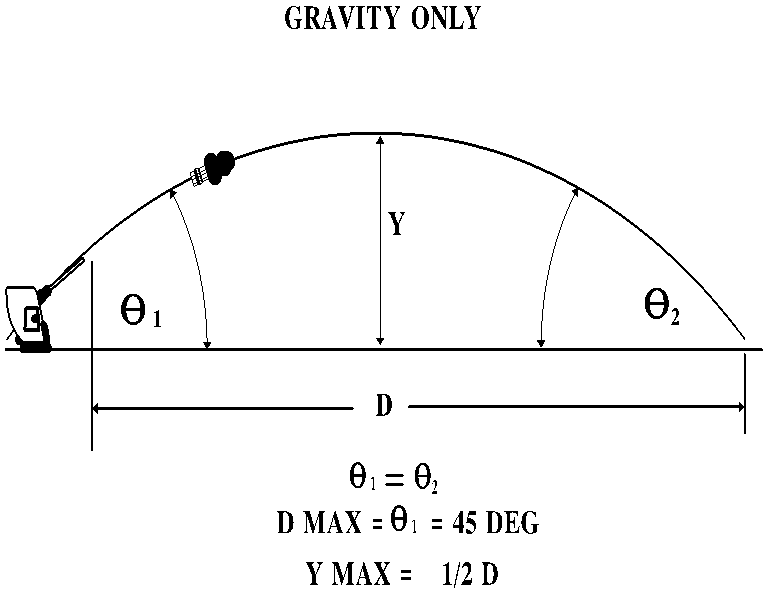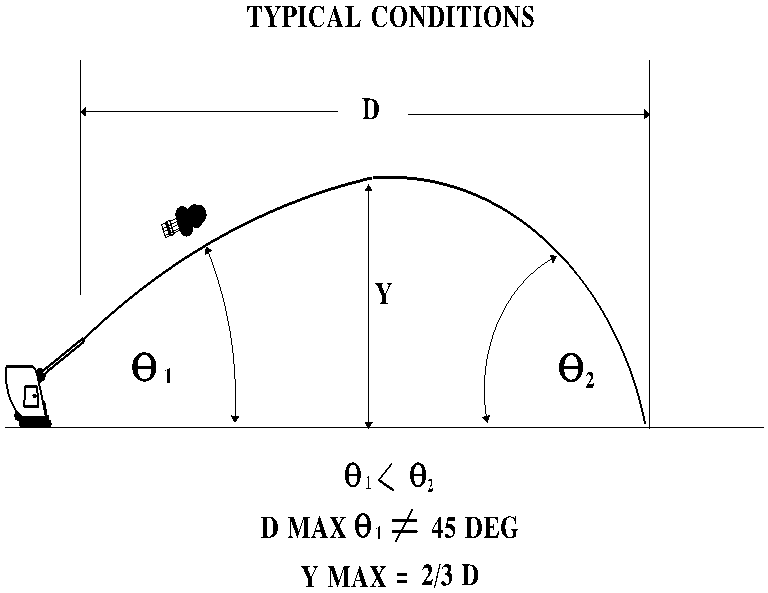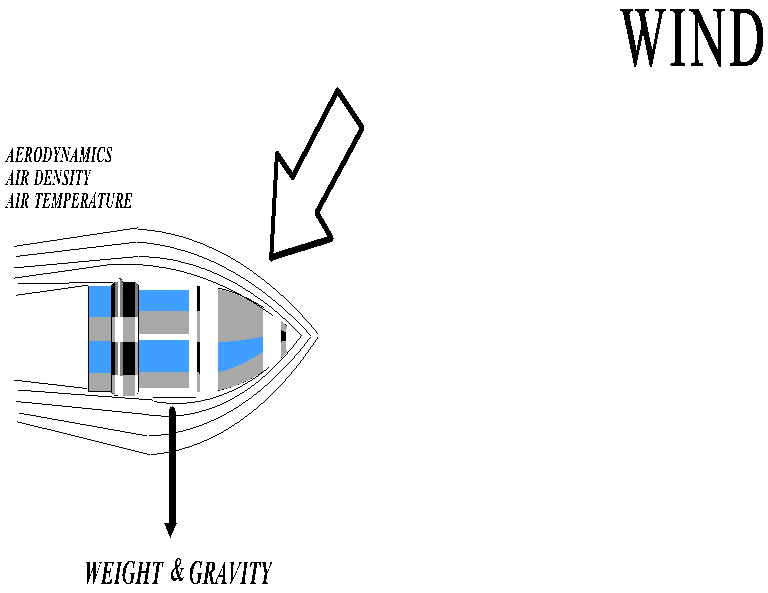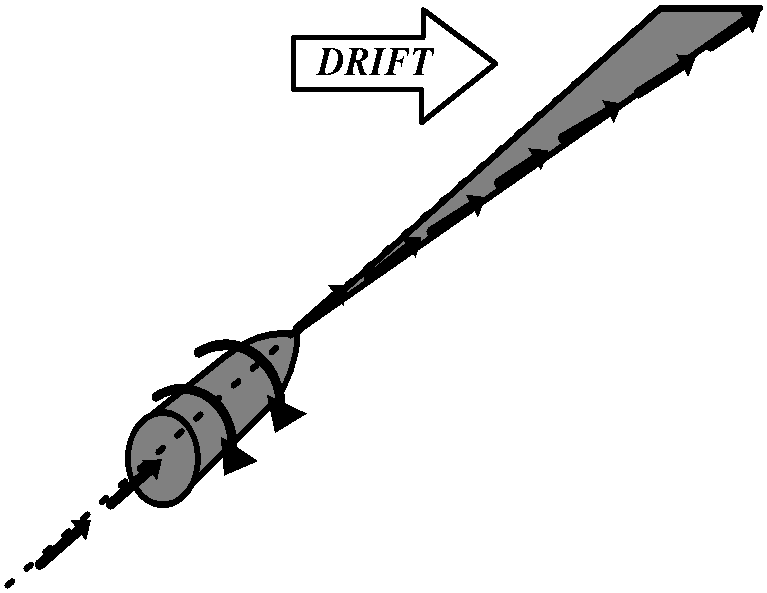####Gunnery Officer

INFORMATION SHEET

INTRODUCTION TO BALLISTICS

Information Sheet Number: 1.6

INTRODUCTION

This lesson is a brief introduction to basic terms and theories of ballistics. It will begin with definitions of terms and ground rules for ballistic calculations to be used in subsequent lessons. Gunnery Officers require a general working knowledge in ballistics, therefore only major contributing forces will be considered and negligent variables will be regarded as constants.

REFERENCES

(a) NAVEDTRA 12970 Principles of Naval Ordnance and Gunnery

INFORMATION

A. DEFINITION OF BALLISTICS

1. The science of the motion of projectile.

B. DIVIDED INTO FOUR BRANCHES

1. Interior ballistics is the branch of the science that deals with the projectile while it is still in the gun.

2. Transitional ballistics is the branch of the science that deals with the motion of the projectile from the time it leaves the muzzle until the only forces acting upon it are due to exterior ballistics.

3. Exterior ballistics is the branch of the science that deals with the projectile after it clears the muzzle and throughout its time of flight.

4. Terminal ballistics is that branch of the science that deals with the impact, and force imparted on the target.

C. FACTORS AFFECTING INTERIOR BALLISTICS

1. Bore erosion caused by:

a. Heat from burning propellant

b. Pressure of powder gases and combustion

c. Chemical action of powder gases and combustion residue

d. Mechanical erosion as projectile passes through the bore

e. Material fatigue

2. Powder index

a. Propelling charges are designed (by shape) to burn in the chamber of the gun in such a manner as to develop maximum projectile velocity without excessive heat, pressure or erosion.

b. The dominant factor used to control burning rate is the surface area per unit weight of propellant, which is determined by the shape of the propellant grain.

3. Initial velocity (IV) - the speed at which the projectile moves through and out the bore, until the rotating bands disengage at the muzzle.

4. Powder temperature - affects the course of propellant combustion and gas pressure build up in the bore. ( Higher powder temperatures result in higher burning rate)

5. Powder weight - amount of powder in casing is directly proportional to initial velocity.

6. Projectile weight - a lighter than standard projectile will have a higher initial velocity imparted to it and vice versa.

7. Other factors

a. Chamber volume - affects pressure buildup.

b. Length of barrel - powder acts on projectile longer thus increasing initial velocity.

c. Barrel temperature - causes powder to burn faster, affecting pressure build up.

d. Rotating bands - impart an aerodynamic rotation, increasing range.

8. Interior ballistics have less effect on a projectile than exterior ballistics, due to the comparatively short time these factors act upon the projectile.

D. TRAJECTORY ANALYSIS

1. No air, no gravity (outer space)

a. The trajectory of a projectile fired under these conditions will have a straight line and will maintain initial velocity indefinitely (unless acted upon by some force).2. Gravity only - no air

a. Perfect parabola shape

b. Maximum ordinate is the altitude at exactly half of the range.

c. Angle of impact will equal elevation angle.

d. Striking velocity will equal initial velocity.

e. The maximum range will only be achieved at an elevation angle of 45 degrees.

3. Air - gravity (normal conditions)

a. No longer a perfect parabola

b. Angle of fall always greater than sight angle.

c. Striking velocity will be less than initial velocity.

d. Maximum ordinate will be approximately two thirds of range.

e. Maximum range is obtained from sight angle of other than 45 degrees.E. EXTERIOR BALLISTIC FACTORS

1. Projectile weight

a. Lighter than standard projectiles will have a higher IV imparted and will travel farther at shorter ranges.

b. Heavier than standard projectiles will develop more momentum and will travel farther at longer ranges.

2. Projectile shape - a better aerodynamic shape reduces turbulence and drag.

3. Wind (obvious effect) - varies with altitude and effect must be considered a constant.

4. Gravity - acts as a constant force.

5. Air density - varies as a function of air temperature, pressure and altitude.

6. Air temperature - affects velocity due to drag coefficient (expressed as a mach number).

7. Initial velocity (IV)

a. The higher the IV the farther the range, however it is not a linear relationship due to an increase in air resistance.

b. A higher IV projectile will have to be heavier than a low IV projectile to achieve the same range.8. Drift

a. Constant deflection to the right.

b. Directly proportional to range, independent of wind; caused by the combined effect of gravity, air resistance and the projectile's clockwise spin.

(1) Spin produces gyroscopic effect on projectile.

(2) Spin is the most significant cause of drift.

(3) A projectile's aerodynamic shape produces an air cushion effect under the projectile itself.